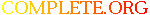Complete.Org: Mailing Lists: Archives: freeciv: January 2004: [Freeciv] Re: D-day# [Freeciv] Re: D-day

[Top] [All Lists]

 To: Wawrzyniec Niewodnicza?ski Cc: freeciv@xxxxxxxxxxx Subject: [Freeciv] Re: D-day From: Lakatos Zoltán Date: Wed, 21 Jan 2004 18:45:15 +0100 (CET)

```=20
Hello!=20
=20
Wawrzyniec Niewodnicza=F1ski <niewod@xxxxxxxxxxxxxxxxxxxx> =EDrta: =20
=20
=20
> Hello  =20
>  =20
> Can you send this my personal to my. This list not allow to =20
send mails with =20
> attachement.  =20
=20
I'm sorry, but my letter contained no attachement. =20
I meant only that, if you know the game Panzer General, =20
in that game you can find a good example, how the idea =20
works in practise. =20
=20
In Freeciv a units step from ship to a grassland or plain =20
reduces its movement points by 1 (movement_cost=3D1). It  =20
seems to me, it would make the game more interesting, if  =20
this (form ship - to plain) movement cost would be larger, =20
say 2.=20
(while from plain - to plain movement cost remains 1)=20
=20
(Can it be implemented through ruleset changes?)=20
=20
bye,=20
Zolt=E1n Lakatos=20
=20

```